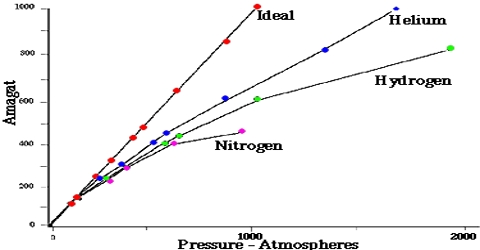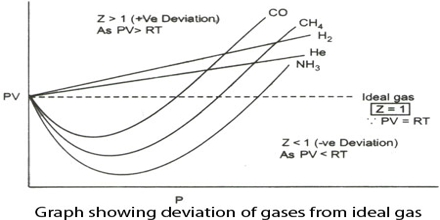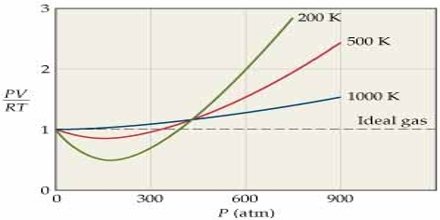# Behavior of Real Gases: The Amagat’s Curves

The behavior of Real Gases: The Amagat’s Curves

The ideal gas law equation PV = nRT is not obeyed by most gases, particularly at high pressures or low temperatures. This was experimentally established by Regnault, Amagat, and others. Only Hydrogen (H₂) and Helium (He) baffle this movement and the curve rises with the rise of Pressure (P) from the very beginning.

The ideal gas law can be derived by means of kinetic molecular theory by making two very significant assumptions that are not proper for real gases:

• There is no attractive force between gas molecules,
• The gas molecules have no volume.

During the nineteenth century, Amagat showed that the product of P and V, i.e., PV, is not quite independent of P, even for gases like H2, O2, N2, etc. This is contrary to Boyle’s law. The nature and magnitude of the deviation are clearly demonstrated from a plot of PV against P as in Figure. These curves are known as Amagati curves. In these curves, PV, an arbitrarily taken as unity at 1 atmosphere and at the temperature of the experiment It a gas obeyed Boyle’s law the curves would be horizontal lines with a constant ordinate equal to RT.From Amagat’s curve, we can see two types of result for real gases.

• If we raise the pressure, the value of PV decreases primarily and then the value starts rising for the gases like- carbon dioxide, nitrogen, oxygen, methane, etc.
• If we raise the pressure, the value of PV starts rising initially for gases like – hydrogen, helium, etc.

It will be observed that the curve for hydrogen shows a steady rise in the value of PV as P increases; for the other gases shown it first passes through a minimum and then rises for still higher values of P. Based on these experiments the following conclusions were made:

(a) the inert gases give curves exactly like hydrogen.

(b) gases which are most easily liquefied deviate to a greater extent from the ideal behavior and

(c) at relatively high temperatures most gases follow curves of the type obtained for hydrogen.

The behavior of real gases may also be described in terms of a compressibility factor Z which is defined as.

Z = PV/RT

For one mole of an ideal gas Z = 1, and is independent of temperature and pressures. At high pressures, the real gases are less compressible. i.e., they have large volume than what is expected from Boyle’s law. At low temperature the gases, particularly the easily liquefiable ones, show more compressibility, i.e., the volume is less than that predicted by Boyle’s law. In general, one may roughly say that the lower the temperature, the higher the compressibility of a gas and the higher the pressure the lower is the compressibility. The effect of temperature on the Z vs. P curve is shown in Figure…Example:

The volume occupied by a certain gas at 600C and 200 atm are 0.124 L. Calculate the compressibility factor of the gas?

Solution: Substituting the data in equation Z = PV/RT; and solving for Z;

Z = (200 x 0.124)/ (0.082 x 333) = 0.908.